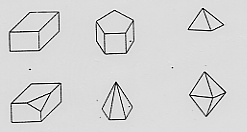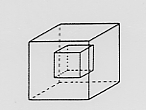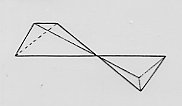Best (IMHO) example from Bloor: Euler's theorem for polyhedra

1752: Euler proposes relation of Verticies, Edges, and Faces:
V - E + F = 2. Polyhedra are defined as "a solid whose faces are polygons."1815: Hessel notes that a cube with a cubic hollow inside does not satisfy Euler's theorem. Polyhedra are redefined as "a surface made up of polygonal faces."1865: Mobius notes that two pyramids joined a tthe vertex also defies Euler's theorem. Polyhedra are redefined as "a system of polygons such that two polygons meet at every edge and where it is possible to get from one face to the other without passing through a vertex."